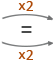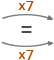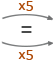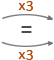## The Four Operations for Fractions

### Adding and Subtracting Fractions

You must NEVER add/subtract unless the denominator is the same for each fraction (if they aren’t the same, you need to find equivalent fractions) and more importantly, NEVER add/subtract the denominators!

Following these steps should help:

Step 1: Make sure the denominators are the same, if not change them.
Step 2: Add/subtract the numerators and put the answer over the denominator.
Step 3: Simplify the final answer.

## Examples

### Example 1

What is 310 + 110 ?

Solution:

310 + 110 = 410 = 25

Notice the answer is NOT 220 and the final answer has been simplified to 25 as both the numerator and denominator have been divided by 2.

### Example 2

What is 7834 ?

Solution:

Make denominators the same:

78 stays as 78

34 changes to: 3468

Now subtract,

7868 = 18

### Adding and Subtracting Mixed Fractions

Step 1: Convert mixed fractions to improper fractions.
Step 2: Make sure the denominators are the same, if not change them.
Step 3: Add/subtract the numerators and put the answer over the denominator.
Step 4: Simplify the final answer/convert back to mixed fraction.

### Example 3

What is 4 25 − 2 37 ?

Solution:

Convert to improper fractions:

4 25 = (4 x 5) + 25 = 225

2 37 = (2 x 7) + 37 = 177

To find a common denominator we find the Lowest Comon Multiple (LCM) of 5 and 7, which is 35.

So,

225 changes to: 22515435

177 changes to: 1778535

Now subtract,

154358535 = 6935

Convert back to mixed fraction:

6935 = 1 3435

### Example 4

What is 1 56 − 2 34 ?

Solution:

Convert to improper fractions:

1 56 = (1 x 6) + 56 = 116

2 34 = (2 x 4) + 34 = 114

To find a common denominator we find the Lowest Comon Multiple (LCM) of 6 and 4, which is 12.

So,

116 changes to: 1162212

114 changes to: 1143312

Add:

22123312 = 5512

Convert back to mixed fraction:

5512 = 4 712